# UpSetR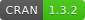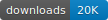## Technique

UpSetR generates static UpSet plots. The UpSet technique visualizes set intersections in a matrix layout and introduces aggregates based on groupings and queries. The matrix layout enables the effective representation of associated data, such as the number of elements in the aggregates and intersections, as well as additional summary statistics derived from subset or element attributes.

For further details about the original technique see the UpSet website. You can also check out the UpSetR shiny app. Here is the source code for the shiny wrapper

## Sample Data

Sample data sets for UpSetR are included in the package and can be loaded like this:

``````movies <- read.csv( system.file("extdata", "movies.csv", package = "UpSetR"), header=T, sep=";" )
mutations <- read.csv( system.file("extdata", "mutations.csv", package = "UpSetR"), header=T, sep = ",")``````

The movie data set created by the GroupLens Lab and curated by Bilal Alsallakh and the mutations data set was originally created by the TCGA Consortium and represents mutations for the 100 most mutated genes in a glioblastoma multiforme cohort.

## Examples

### Vignettes

There are currently four vignettes that explain how to use the features included in the UpSetR package: * Basic Usage * Queries * Attribute Plots * Set Metadata

### Demo

A view of the UpSet plot with additional plots based on elements in the intersections.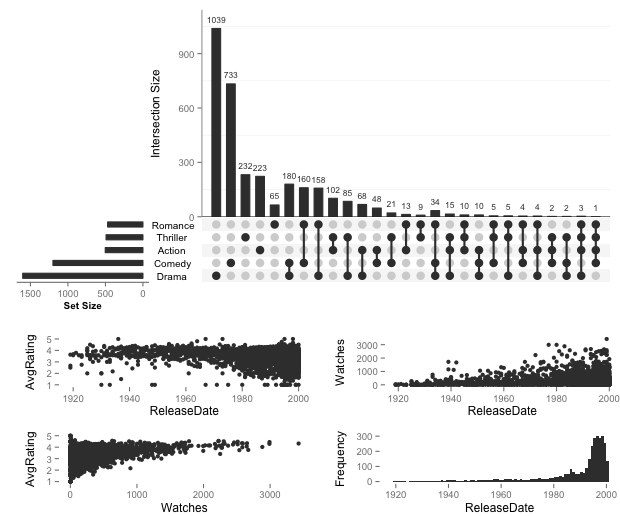Image

``````upset(movies,attribute.plots=list(gridrows=60,plots=list(list(plot=scatter_plot, x="ReleaseDate", y="AvgRating"),
list(plot=scatter_plot, x="ReleaseDate", y="Watches"),list(plot=scatter_plot, x="Watches", y="AvgRating"),
list(plot=histogram, x="ReleaseDate")), ncols = 2))``````

A view of UpSetR mimicking the plot published by Lex & Gehlenborg http://www.nature.com/nmeth/journal/v11/n8/abs/nmeth.3033.html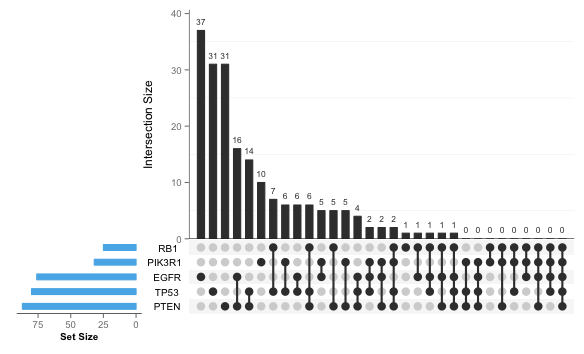image

``````upset(mutations, sets = c("PTEN", "TP53", "EGFR", "PIK3R1", "RB1"), sets.bar.color = "#56B4E9",
order.by = "freq", empty.intersections = "on")``````

An example using two set queries (war movies and noir movies) along with attribute plots comparing the average rating (top) and average rating vs the number of times the movies have been watched (bottom).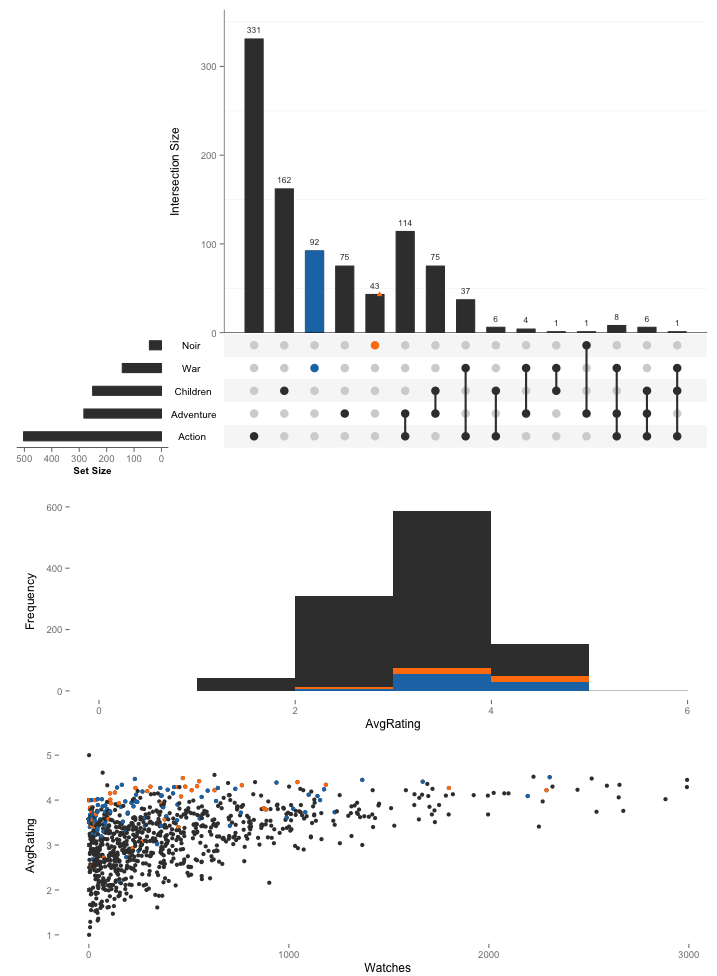image

``````upset(movies, attribute.plots=list(gridrows = 100, ncols = 1,
plots = list(list(plot=histogram, x="AvgRating",queries=T),
list(plot = scatter_plot, y = "AvgRating", x = "Watches", queries = T))),
sets = c("Action", "Adventure", "Children", "War", "Noir"),
queries = list(list(query = intersects, params = list("War"), active = T),
list(query = intersects, params = list("Noir"))))``````

Install the latest released version from CRAN

``install.packages("UpSetR")``

Download the latest development code of UpSetR from GitHub using devtools with

``devtools::install_github("hms-dbmi/UpSetR")``

## Citation

If you use UpSetR in a paper, please cite:

Alexander Lex, Nils Gehlenborg, Hendrik Strobelt, Romain Vuillemot, Hanspeter Pfister,
UpSet: Visualization of Intersecting Sets,
IEEE Transactions on Visualization and Computer Graphics (InfoVis '14), vol. 20, no. 12, pp. 1983–1992, 2014.
doi:10.1109/TVCG.2014.2346248